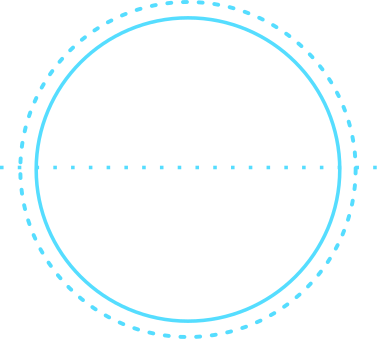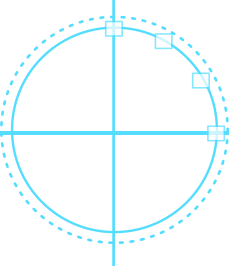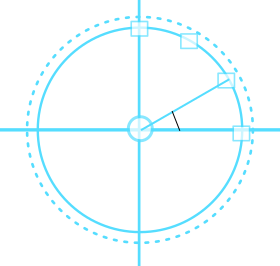# Happi Pi DayWritten by
Published on
Modified on
CategoryFor as long as I can remember, I was both aware and unaware of Pi. And I took it for granted really. 3.14. That's all that was known from this point of view. Didn't know why or how or in what dimension it made sense, but it followed me around during the school years. So it's only fitting that on this Pi Day, we take a look at what exactly (or as close as can be explained) Pi is, and how I have used it in my own programming to help solve some of life's problems.

### What is PI?

Pi is an amazing thing. And it's kind of hard to wrap one's head around. But essentially, if you divide a circle's circumference by its diameter, it will always be equal to the value of Pi, of which "we" know 3 digits mainly. And by "we", I mean most people, as there are people out there working to compute quadrillionth values of it. But that sounds too simple of an explanation. And if I was a mathematician and had about 5 or 6 chalkboards, I'd be able to explain the whole thing better. But the best way to approach it is to think of it as this. That as a circle grows in circumference, it's diameter will expand by 1/3 of that measure.

simple, yet profound

### The last time I used Pi

I've only used Pi on one occasion in my programming life and it was very unexpected and sort of required some research on my part. Circles are fickle things and you can't do too much without throwing a Pi around here or there. The following is the account of that fateful program and the resulting circular shape that developed.

#### The clock

The goal for this project was to render a clock from scratch using JavaScript. Sounded incredibly simple when I had the thought, mainly because the following would render a nice round shape without too much effort.

``````
<style>
.clock
{
width:200px;
height:200px;
border:solid  1px #555;
margin:0 auto;
}
</style>
```
```

Simple enough right? The browser does all of the math work, and I just have to sit back and enjoy the results. Except that's not a clock. It's just a circle. Next up came adding the clock elements, and this is where things got wild and mathy.To simplify things, a clock has 12 points equally spaced from each other following the curve of the clock body. The next task is to position each of those 12 elements in their corresponding X and Y coordinates. And given the circumference and diameter of a circle, we can indeed figure out the X and Y coordinates on the circles periphery.

In order to do that, we'll need to know a few things. The number of points along the circle, from zero to infinite. The angle that each of these lines makes. And the radius, which we know because it is half the length of the diameter.

``````
function drawHours(el)
{
var numpoints = 12;
var step_angle = 360 / numpoints;

for(var i = 0; i < numpoints; i++)
{
var angle = (90 - (i * step_angle));
var angleradians = (Math.PI / 180) * angle;
drawHour(i, x, y, el);    // renders the UI for the hour
}
}
```
```The magic happens in the GetXCoordinate() and GetYCoordinate functions. For this example, a unit circle was assumed with center landing on [0, 0]. And the Cartesian Coordinates can be calculated as the following:

```Y = sin((pi/180) * angle) * radius;
X = cos((pi/180) * angle) * radius;
```

Which translates into code as the following:

``` function GetYCoordinate(radius, angle) { var result = parseInt(radius) - Math.floor(Math.sin((Math.PI / 180) * angle) * radius); return result; } function GetXCoordinate(radius, angle) { var result = Math.floor(Math.cos((Math.PI / 180) * angle) * radius) + parseInt(radius); return result; } ```

And like that, a clock was born. It's a very simple formula for the most part and didn't require too much coding work, but getting to know the circle and the concept of pi took days and dreams. And this appreciation grew out of that, that wasn't there before. Now when I look at a clock, I realize that those points aren't just randomly placed there. When a clocks hand moves, it isn't just a random increment that it moves by. It was just a circle one minute, and a whole hidden mechanism the next.

### Looking forward to all the circles

So on this day, eat a slice of pizza, or of pie (at a discount hopefully) and remember that all the circles you see have a circumference that is around 3 times the size of the diameter and ponder that thought. Happy Pi Day!Walter Guevara is a software engineer, startup founder and currently teaches programming for a coding bootcamp. He is currently building things that don't yet exist.